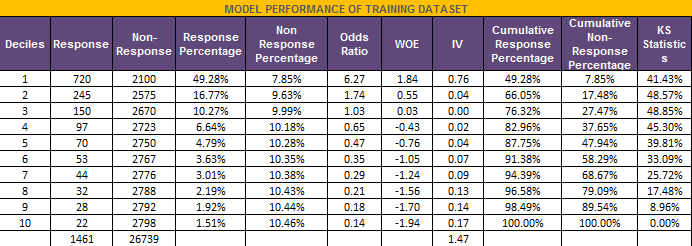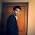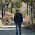# Detecting and solving problem of Outlier

Detecting Outliers

There are two simple ways you can detect outlier problem :

1. Box Plot Method

If a value is higher than the 1.5*IQR above the upper quartile (Q3), the value will be considered as outlier. Similarly, if a value is lower than the 1.5*IQR below the lower quartile (Q1), the value will be considered as outlier.
QR is interquartile range. It measures dispersion or variation. IQR = Q3 -Q1.
Lower limit of acceptable range = Q1 - 1.5* (Q3-Q1)
Upper limit of acceptable range = Q3 + 1.5* (Q3-Q1)
Some researchers use 3 times of interquartile range instead of 1.5 as cutoff. If a high percentage of values are appearing as outliers when you use 1.5*IQR as cutoff, then you can use the following rule
Lower limit of acceptable range = Q1 - 3* (Q3-Q1)
Upper limit of acceptable range = Q3 + 3* (Q3-Q1)
SAS Macro for identifying outliers

2. Standard Deviation Method

If a value is higher than the mean plus or minus three Standard Deviation is considered as outlier. It is based on the characteristics of a normal distribution for which 99.87% of the data appear within this range.
Acceptable Range : The mean plus or minus three Standard Deviation

This method has several shortcomings :
1. The mean and standard deviation are strongly affected by outliers.
2. It assumes that the distribution is normal (outliers included)
3. It does not detect outliers in small samples

3. Percentile Capping (Winsorization)

In layman's terms, Winsorization (Winsorizing) at 1st and 99th percentile implies values that are less than the value at 1st percentile are replaced by the value at 1st percentile, and values that are greater than the value at 99th percentile are replaced by the value at 99th percentile. The winsorization at 5th and 95th percentile is also common.

Which of the above 3 methods is more robust?

The box-plot method is less affected by extreme values as compared to Standard Deviation method. If the distribution is skewed, the box-plot method fails. The Winsorization method is a industry standard technique to treat outliers. It works well. In contrast, box-plot and standard deviation methods are traditional methods to treat outliers. They were designed and used when researchers used to do calculations using pen and paper.

How to overcome problem of outliers
1. Drop extreme values - SAS Code
2. Capping - Replacing extreme values with the acceptable limits as shown in the above 3 methods.
SAS Code for Percentile Capping / Winsorization :

/*************************************************
Percentile Capping / Winsorize macro
*input = dataset to winsorize;
*output = dataset to output with winsorized values;
*class = grouping variables to winsorize;
* Specify "none" in class for no grouping variable;
*vars = Specify variable(s) in which you want values to be capped;
*pctl = define lower and upper percentile - acceptable range;
**************************************************/

%macro pctlcap(input=, output=, class=none, vars=, pctl=1 99);

%if &output = %then %let output = &input;

%let varL=;
%let varH=;
%let xn=1;

%do %until (%scan(&vars,&xn)= );
%let token = %scan(&vars,&xn);
%let varL = &varL &token.L;
%let varH = &varH &token.H;
%let xn=%EVAL(&xn + 1);
%end;

%let xn=%eval(&xn-1);

data xtemp;
set &input;
run;

%if &class = none %then %do;

data xtemp;
set xtemp;
xclass = 1;
run;

%let class = xclass;
%end;

proc sort data = xtemp;
by &class;
run;

proc univariate data = xtemp noprint;
by &class;
var &vars;
output out = xtemp_pctl PCTLPTS = &pctl PCTLPRE = &vars PCTLNAME = L H;
run;

data &output;
merge xtemp xtemp_pctl;
by &class;
array trimvars{&xn} &vars;
array trimvarl{&xn} &varL;
array trimvarh{&xn} &varH;

do xi = 1 to dim(trimvars);
if not missing(trimvars{xi}) then do;
if (trimvars{xi} < trimvarl{xi}) then trimvars{xi} = trimvarl{xi};
if (trimvars{xi} > trimvarh{xi}) then trimvars{xi} = trimvarh{xi};
end;
end;
drop &varL &varH xclass xi;
run;

%mend pctlcap;

%pctlcap(input=test, output=result, class=none, vars = X1 X2 X7, pctl=1 99);

4. Weight of Evidence

Weight of Evidence for a category = log (% events / % non-events) in the category

Weight of Evidence was originated from logistic regression technique. It tells the predictive power of an independent variable in relation to the dependent variable. It is calculated by taking the natural logarithm (log to base e) of division of % of non-events and % of events.

Outlier Treatment with Weight Of Evidence : Outlier classes are grouped with other categories based on Weight of Evidence (WOE)Outlier Detection : WOE
How it works -

Suppose you have a income variable and outlier values are more than 500 million dollars. These values would be grouped to a class of (let's say 250-500 million dollars). Later, instead of using the raw values, we would be using WOE scores of each classes.Related PostsDeepanshu founded ListenData with a simple objective - Make analytics easy to understand and follow. He has over 8 years of experience in data science. During his tenure, he has worked with global clients in various domains like Banking, Insurance, Telecom and Human Resource.

6 Responses to "Detecting and solving problem of Outlier"
1.How WOE is used to detect and solve outlier problem?

1.Outlier classes are grouped with other categories based on Weight of Evidence (WOE). For example, you have a income variable and outlier values are more than 500 million dollars. These values would be grouped to a class of (let's say 250-500 million dollars). Later, instead of using the raw values, we would be using WOE scores of each classes. Hope it helps. Thanks!

2.WOE is used only in the logistic regression only ? Can you please confirm which outlier treatment used for the linear regression only

1.WOE is more common for binary dependent variable. It was originated from the logistic regression technique. You can use percentile capping (aka Winsorization) for treating outliers in linear regression. It can also be used in logistic regression. It is not restricted to linear regression.

3.Thanks for wonderful article!! I love all articles that you post.
Can you explain one we always look for Q1- 1.5 QTR/Q3+1.5QTR? I mean why 1.5 factor we are using not 1,2,2.5

4.There is nice explanation on Wikipedia under article "Interquartile range":
https://en.wikipedia.org/wiki/Interquartile_range

Next → ← Prev
Love this Post? Spread the Word!
Share